Tapping into mathematics

Start this free course now. Just create an account and sign in. Enrol and complete the course for a free statement of participation or digital badge if available.

Free course

# 7.6 Consolidation

You have probably learnt quite a lot about your calculator by now. So this may be a good time to pause and consolidate that knowledge. Speaking mathematics aloud and explaining concepts to somebody else are good ways to do this.

## Exercise 15: Speakeasy

This exercise is to give you practice in saying as well as doing mathematics. First set your calculator to 4 places of decimals. Next, complete the table by noting in the second column how you would say the mathematical expressions out loud. Finally, note down their values in the third column.

MathsSayValue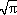The square root of pi1.7725
29
(2.5 × 7.2)− 1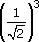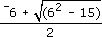PressSayKey sequenceValueThe square root of pi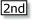[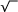][]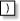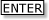1.7725
29Two to the power 92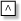9512.0000
(2.5 × 7.2)− 1The reciprocal of (2.5 times 7.2), or 2.5 times 7.2 all to the power of minus 1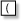2.5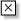7.2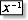.0556The reciprocal of the square root of 2 to the power 3, or one over root 2, all cubed[] 23.3536Minus 6 plus the square root of (6 squared minus 15), all divided by two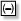6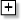[] 6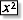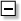15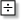2.7087

Notice the importance of brackets in the last example. An extra pair of brackets is necessary in the key sequence to ensure that everything is divided by 2.

You may like to reset your calculator to FLOAT, before using it again.

## Exercise 16: Explaining to others

Depending on your previous experience, this may have been the first time you have come across the mathematical terms ‘square’, ‘square root’, ‘reciprocal’ and ‘power’. Do you feel you could now explain what they mean to someone else who is unfamiliar with these terms?

Using your own words, try to write a few notes in your Learning Journal [Tip: hold Ctrl and click a link to open it in a new tab. (Hide tip)] on each term as if you were explaining the meanings to someone else. If you are still hazy about the meaning of the terms, then try to be clear about what it is that you do not understand.

Many different answers are possible, but you should have included something equivalent to the following.

The ‘square’ (key) of a number is the number multiplied by itself, for example 42=4×4=16.

The ‘square root’ ( []) of a positive number is the number whose square gives the original number, for example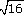=4.

The ‘reciprocal’ (key) of a number is 1 divided by the number, for example the reciprocal of 2=.

The ‘power’ (key) is the number of times a number is multiplied by itself, for example 23 is 2 to the power 3, which is 2 × 2 × 2.

You might like to try out your explanations on someone else.

You have started to unlock some of the power of your calculator by exploring a few of its functions. You have looked at some examples of everyday calculations and explored techniques for handling very large and very small numbers. You have also started to use some mathematical language. This is only the first step and is intended to give you an initial appreciation of the calculator as a mathematical tool.

MU120_4MCAL